# Partial derivative

## Definition at a point

### Generic definition

Suppose$f$ is a function of more than one variable, where$x$ is one of the input variables to$f$. Fix a choice$x = x_0$ and fix the values of all the other variables. The partial derivative of$f$ with respect to$x$, denoted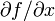$\partial f/\partial x$, or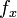$f_x$, is defined as the derivative at$x_0$ of the function that sends$x$ to$f$ at$x$ for the same fixed choice of the other input variables.

### For a function of two variables

Suppose$f$ is a real-valued function of two variables$x,y$, i.e., the domain of$f$ is a subset of$\R^2$. We define the partial derivatives as follows:

• Partial derivative with respect to$x$: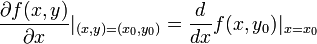$\frac{\partial f(x,y)}{\partial x}|_{(x,y) = (x_0,y_0)} = \frac{d}{dx}f(x,y_0)|_{x = x_0}$

In words, it is the derivative at$x = x_0$ of the function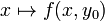$x \mapsto f(x,y_0)$.

This partial derivative is also denoted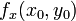$f_x(x_0,y_0)$ or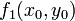$f_1(x_0,y_0)$.

• Partial derivative with respect to$y$: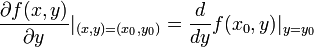$\frac{\partial f(x,y)}{\partial y}|_{(x,y) = (x_0,y_0)} = \frac{d}{dy}f(x_0,y)|_{y = y_0}$

In words, it is the derivative at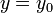$y = y_0$ of the function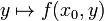$y \mapsto f(x_0,y)$.

This partial derivative is also denoted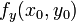$f_y(x_0,y_0)$ or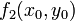$f_2(x_0,y_0)$.

### For a function of multiple variables

Fill this in later